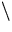Next: Activity Up: Pointers Previous: Pointer Operators

## Calling Functions with Arrays

When an array is an argument to a function, only the address of the first element of the array is passed, not a copy of the entire array. There are three ways to declare a parameter that is to receive an array pointer. First it can be declared as an array of the same type and size as that used to call the function, as shown here:

Example
#include <iostream.h>
// calling functions with arrays
void display(int num);
main()
{
int t,i;
for(i=0;i<10;++i)t[i]=i;
display(t);
return 0;
}
void display(int num)
{
int i;
for(i=0;i<10;i++) cout <<num[i]<<' ';
}

Even though the parameter num is declared to be an integer array of 10 elements, the C++ compiler will automatically convert it to an integer pointer. This is necessary because no parameter can actually receive an entire array. A second way to declare an array parameter is to specify it as an unsized array as shown here:

void display(int num[])
{
int i;
for(i=0; i<10; i++) cout << num[i] <<' ';
}

Here, num is declared to be an integer array of unknown size. C++ provides no array boundary checks, the actual size of the array is irrelevant to the parameter.

The final way that num can be declared is as a pointer. This method is most commonly used in professionally written C++ programs. Here is an example:

void display(int *num)
{
int i;
for(i=0;i<10;i++) cout<<num[i]<<' ';
}

On the other hand, an array element used as an argument is treated like any other simple variable. For example, the preceding program could also be written without passing the entire array, as shown here:

#include <iostream.h>
void display(int num);
main()
{
int t,i;
for(i=0;i<10;++i)t[i]=i;
for(i=0;i<10;i++)display(t[i]);
return 0;

}

void display(int num)
{
cout <<num<<' ';
}
As you can see, the parameter to display() is of type int. It is not relevant that display() is called using an array element, because only that one value of the array is used.

It is important to remember that when an array is used as a function argument, its address is passed to a function. This means that the code inside the function will be operating on, and potentially altering, the actual content of the array used to call the function. For example the following example the program examine the function cube() which converts the value of each element in the array into its cube.

Example #include <iostream.h>
void cube(int *n, int num);
main()
{
int i, nums;
for(i=0;i<10;i++) nums[i]=i+1;
cout <<"Original contents:"; for(i=0;i<10;i++) cout << nums[i] << ' '; cout << 'n';
cube(nums, 10);
cout <<"Altered contents :";
for(i=0;i<10;i++) cout<<nums[i]<<' ';
return 0;
}
void cube(int *n, int num)
{
while(num) {
*n=*n * *n * *n;
num-; }
}

After the call to cube(), the contents of array nums in main will be cubes of its original values. That is the value of elements of nums have been modified by the statements cube(), because n points to nums.Next: Activity Up: Pointers Previous: Pointer Operators
Yousef Haik
2/23/1998